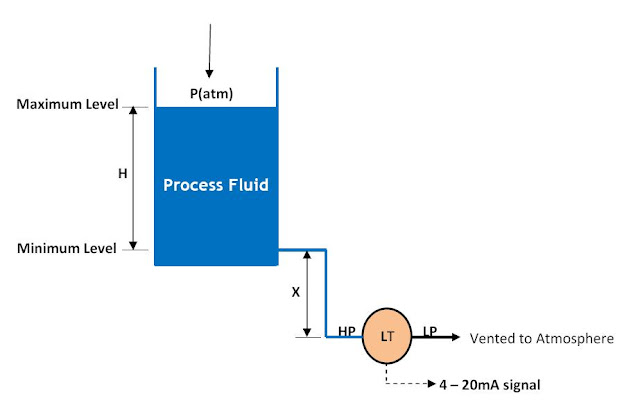Zero Suppression Calibration in Level Measurement ~ Learning Instrumentation And Control Engineering Learning Instrumentation And Control Engineering

### Zero Suppression Calibration in Level Measurement

Custom Search

In some cases of level measurement in open tanks, the level transmitter has to be installed some distance below the base of the tank as shown below:Zero Suppression in Open Tank Level Measurement InstallationFrom the diagram above, it can be seen that when the tank level is minimum (H = 0), the liquid in the high pressure impulse line exerts a constant pressure (P = X*S.G) on the high pressure side.
When the liquid level is at a maximum, H meters, pressure on the high pressure side of the transmitter will be:
P(high) = S.G*H + S.G*X + P(atm)
P(low)= P(atm)
 Differential Pressure, ΔP = P(high) – P(low) =  S.G * H + S.G * X

With this, the transmitter output is now proportional to both S.G*H and S.G* X. To make the transmitter output proportional to only S.G*H, we suppress the S.G*X part of the transmitter output by negatively biasing the transmitter by –S.G*X.
This implies that the range of level measurement will be between S.G*X to (S.G*H + S.G*X.) This procedure is called Zero Suppression and is done when calibrating the level or DP transmitter.

Consider the example below:
Suppose the maximum level of liquid in the tank is 40ft, the Specific gravity of the liquid, S.G = 0.9. If the level transmitter for level measurement is mounted 6ft below the base of the tank then we can calibrate the level transmitter as follows:
H = 40ft = 40*12 = 480in
X = 6ft = 6*12 = 72in
S.G = 0.9
Differential pressure between the level transmitter HP and LP when tank is full is:
= S.G*H + S.G*X = 0.9*480 + 0.9*72 = 496.8 in wc
When tank is empty (H = 0),
Differential pressure = S.G*X = 0.9*72 = 64.8 in wc.
The transmitter is then calibrated as shown below:
<![endif]-->At 0% minimum tank level (H = 0) 4mA = 64.8in wc and at 100% maximum tank level (H = 40ft) 20mA = 496.8 in wc. Note that the same calibration method above is used if the installation is a dry leg.

Continue to the next sections on Level Measurement: